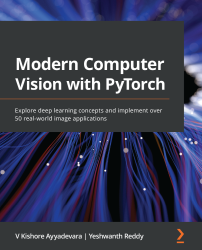•#### Modern Computer Vision with PyTorch#### Overview of this book

Deep learning is the driving force behind many recent advances in various computer vision (CV) applications. This book takes a hands-on approach to help you to solve over 50 CV problems using PyTorch1.x on real-world datasets. You’ll start by building a neural network (NN) from scratch using NumPy and PyTorch and discover best practices for tweaking its hyperparameters. You’ll then perform image classification using convolutional neural networks and transfer learning and understand how they work. As you progress, you’ll implement multiple use cases of 2D and 3D multi-object detection, segmentation, human-pose-estimation by learning about the R-CNN family, SSD, YOLO, U-Net architectures, and the Detectron2 platform. The book will also guide you in performing facial expression swapping, generating new faces, and manipulating facial expressions as you explore autoencoders and modern generative adversarial networks. You’ll learn how to combine CV with NLP techniques, such as LSTM and transformer, and RL techniques, such as Deep Q-learning, to implement OCR, image captioning, object detection, and a self-driving car agent. Finally, you'll move your NN model to production on the AWS Cloud. By the end of this book, you’ll be able to leverage modern NN architectures to solve over 50 real-world CV problems confidently.
PrefaceSection 1 - Fundamentals of Deep Learning for Computer VisionFree Chapter
Artificial Neural Network FundamentalsPyTorch FundamentalsBuilding a Deep Neural Network with PyTorchSection 2 - Object Classification and DetectionIntroducing Convolutional Neural NetworksTransfer Learning for Image ClassificationPractical Aspects of Image ClassificationBasics of Object DetectionImage SegmentationApplications of Object Detection and SegmentationSection 3 - Image ManipulationAutoencoders and Image ManipulationImage Generation Using GANsSection 4 - Combining Computer Vision with Other TechniquesTraining with Minimal Data PointsCombining Computer Vision and NLP TechniquesCombining Computer Vision and Reinforcement LearningMoving a Model to ProductionUsing OpenCV Utilities for Image AnalysisOther Books You May EnjoyBuilding a Deep Neural Network with PyTorch

In the previous chapter, we learned how to code a neural network using PyTorch. We also learned about the various hyperparameters that are present in a neural network, such as its batch size, learning rate, and loss optimizer. In this chapter, we will shift gears and learn how to perform image classification using neural networks. Essentially, we will learn how to represent images and tweak the hyperparameters of a neural network to understand their impact.

For the sake of not introducing too much complexity and confusion, we only covered the fundamental aspects of neural networks in the previous chapter. However, there are many more inputs that we tweak in a network while training it. Typically, these inputs are known as hyperparameters. In contrast to the parameters in a neural network (which are learned during training), these inputs...